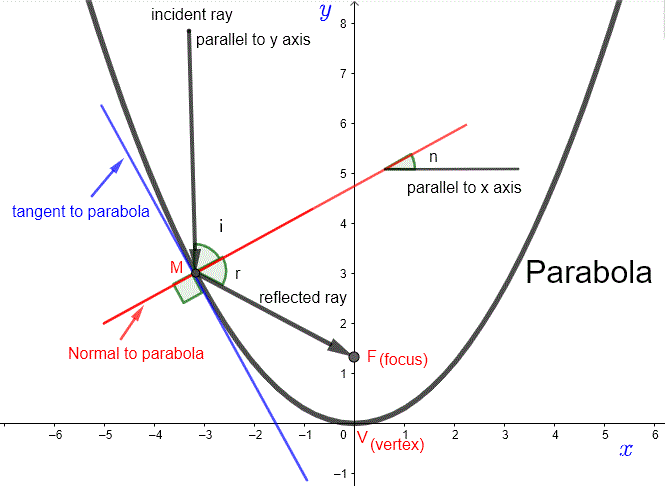# How Parabolic Antennas work?

It is shown, mathematically, how a parabolic antenna work by reflecting electromagnetic rays parallel to its axis onto the focus as a receiver. Also a feed of electromagnetic waves at the focus of a parabolic antenna will emit rays that are reflected by the susrface of the parabola in the direction parallel to its axis.

## Parabolic Antenna

An electromagnetic ray parallel to the axis of the parabola (in this case the y axis) is incident on the inner surface of the parabola; see figure below.Point M is the point at which the ray hits the parabolic dish. i is the angle made by the incident ray and the normal (in red) which is perpendicular to the tangent (in blue) to the parabola at point M(a,b) . r is the angle made by the reflected ray and the normal. According to the laws of reflection, angles i and r are equal.
We will show that all reflected rays due to incident rays, at different positions, intercept the axis of the parabola y axis) at the same.

We first calculate the slope of the tangent mt in order to obtain the slope of the normal mn .
The slope of the tangent is given by the value of the first derivative at point M(a,b) .

For a given parabolic reflector (or dish), the equation of the parabola can be written as
y = x2 / (4 f)
where f is a constant.
see Find The Focus of Parabolic Dish Antennas for more details.

The first derivative is given by
y ' = x / (2 f)

The slope of the tangent at point M(a , b) is given by
mt = a / (2 f)

The slope of the tangent mt and the slope of the normal mn at point M are related by
mt × mn = -1 .

Substitute mt = a / (2 f) in the above to obtain
mn = -2f / a

Let n be the angle made by the normal and the x axis.
tan(n) = -2f / a (relationship between angles and slopes).

The angle made by the reflected ray and the x axis is equal to
n - r = n - i, since r = i

Let mr be the slope of the reflected ray.
Hence mr = tan(n - i).

also i + n = 90o.
Combine the last two to obtain
mr = tan(2 n - 90)

tan(2 n - 90) can be written as
tan(2 n - 90) = - cot(2 n)
= - 1 / tan(2 n) = - (1-tan2 n)/(2*tan n)
.

Hence
mr = - (1-tan2 n)/(2 tan n)

Substitute tan(n) = - 2f / a into the above and simplify to obtain
mr = (a2 - 4f2) / 4(f a)

Now that we have the slope of the reflected ray, let us find an equation for the line through point M(a , b) and with the same slope as the incident ray. In point slope form the equation is given by
y - b = mr (x - a)

The y intercept of the above line is given by
y-intercept = b - a × mr

Since point M is on the parabola, b = a2 / 4f

Substitute b and mr in the expression that gives the y-intercept to obtain
y-intercept = b - a mr
= a2 / 4f - a (a2 - 4f2) / 4(f a)

= f.
The y intercept is equal to f which is constant for a given parabolic reflector (or dish). It does not depend on the position of the incident ray. Hence all the reflected rays pass through the same point (0 , f) called the focus and f is the focal distance. In practical situations a device is placed at the focus (0 , f) to collect all the incoming electromagnetic energy.

Note We have used algebra, trigonometry, calculus, physics laws of reflection to explain how a parabolic reflector work. This is a good example of how are mathematics applied in engineering and physics to solve real life problems.
Parabolic antennas may be used in different situations as explained below.

## Parabolic Antenna in Receiving Mode

In receiving mode, electromagnetic rays incoming from space are reflected at the surface of the parabolic antenna and they then converge to the same point called focus.## Parabolic Antenna in Emiting Mode

In emitting mode, electromagnetic rays radiated from the focus are reflected at the surface of the parabolic antenna and they are then transmitted as plane plaves into space.## Exercises

Find the focus of each of the parabolas whose equations are given below
1) y = 4 x2.
2) y = x2 / 8.
3) y = 0.12 x2.